# 8.1 Graphs of the sine and cosine functions  (Page 8/13)

 Page 8 / 13

For the equation $\text{\hspace{0.17em}}A\text{\hspace{0.17em}}\mathrm{cos}\left(Bx+C\right)+D,$ what constants affect the range of the function and how do they affect the range?

The absolute value of the constant $\text{\hspace{0.17em}}A\text{\hspace{0.17em}}$ (amplitude) increases the total range and the constant $\text{\hspace{0.17em}}D\text{\hspace{0.17em}}$ (vertical shift) shifts the graph vertically.

How does the range of a translated sine function relate to the equation $\text{\hspace{0.17em}}y=A\text{\hspace{0.17em}}\mathrm{sin}\left(Bx+C\right)+D?$

How can the unit circle be used to construct the graph of $\text{\hspace{0.17em}}f\left(t\right)=\mathrm{sin}\text{\hspace{0.17em}}t?$

At the point where the terminal side of $\text{\hspace{0.17em}}t\text{\hspace{0.17em}}$ intersects the unit circle, you can determine that the $\text{\hspace{0.17em}}\mathrm{sin}\text{\hspace{0.17em}}t\text{\hspace{0.17em}}$ equals the y -coordinate of the point.

## Graphical

For the following exercises, graph two full periods of each function and state the amplitude, period, and midline. State the maximum and minimum y -values and their corresponding x -values on one period for $\text{\hspace{0.17em}}x>0.\text{\hspace{0.17em}}$ Round answers to two decimal places if necessary.

$f\left(x\right)=2\mathrm{sin}\text{\hspace{0.17em}}x$

$f\left(x\right)=\frac{2}{3}\mathrm{cos}\text{\hspace{0.17em}}x$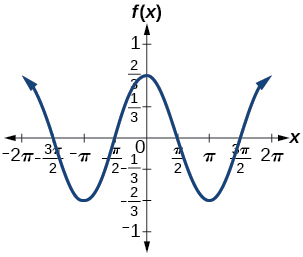amplitude: $\text{\hspace{0.17em}}\frac{2}{3};\text{\hspace{0.17em}}$ period: $\text{\hspace{0.17em}}2\pi ;\text{\hspace{0.17em}}$ midline: $\text{\hspace{0.17em}}y=0;\text{\hspace{0.17em}}$ maximum: $\text{\hspace{0.17em}}y=\frac{2}{3}\text{\hspace{0.17em}}$ occurs at $\text{\hspace{0.17em}}x=0;\text{\hspace{0.17em}}$ minimum: $\text{\hspace{0.17em}}y=-\frac{2}{3}\text{\hspace{0.17em}}$ occurs at $\text{\hspace{0.17em}}x=\pi ;\text{\hspace{0.17em}}$ for one period, the graph starts at 0 and ends at $\text{\hspace{0.17em}}2\pi$

$f\left(x\right)=-3\mathrm{sin}\text{\hspace{0.17em}}x$

$f\left(x\right)=4\mathrm{sin}\text{\hspace{0.17em}}x$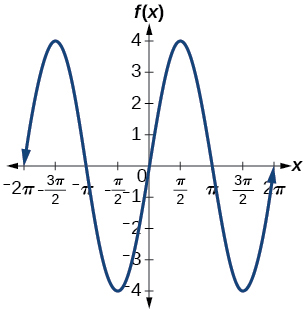amplitude: 4; period: $\text{\hspace{0.17em}}2\pi ;\text{\hspace{0.17em}}$ midline: $\text{\hspace{0.17em}}y=0;\text{\hspace{0.17em}}$ maximum $\text{\hspace{0.17em}}y=4\text{\hspace{0.17em}}$ occurs at $\text{\hspace{0.17em}}x=\frac{\pi }{2};\text{\hspace{0.17em}}$ minimum: $\text{\hspace{0.17em}}y=-4\text{\hspace{0.17em}}$ occurs at $\text{\hspace{0.17em}}x=\frac{3\pi }{2};\text{\hspace{0.17em}}$ one full period occurs from $\text{\hspace{0.17em}}x=0\text{\hspace{0.17em}}$ to $\text{\hspace{0.17em}}x=2\pi$

$f\left(x\right)=2\mathrm{cos}\text{\hspace{0.17em}}x$

$f\left(x\right)=\mathrm{cos}\left(2x\right)$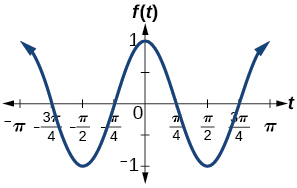amplitude: 1; period: $\text{\hspace{0.17em}}\pi ;\text{\hspace{0.17em}}$ midline: $\text{\hspace{0.17em}}y=0;\text{\hspace{0.17em}}$ maximum: $\text{\hspace{0.17em}}y=1\text{\hspace{0.17em}}$ occurs at $\text{\hspace{0.17em}}x=\pi ;\text{\hspace{0.17em}}$ minimum: $\text{\hspace{0.17em}}y=-1\text{\hspace{0.17em}}$ occurs at $\text{\hspace{0.17em}}x=\frac{\pi }{2};\text{\hspace{0.17em}}$ one full period is graphed from $\text{\hspace{0.17em}}x=0\text{\hspace{0.17em}}$ to $\text{\hspace{0.17em}}x=\pi$

$f\left(x\right)=2\text{\hspace{0.17em}}\mathrm{sin}\left(\frac{1}{2}x\right)$

$f\left(x\right)=4\text{\hspace{0.17em}}\mathrm{cos}\left(\pi x\right)$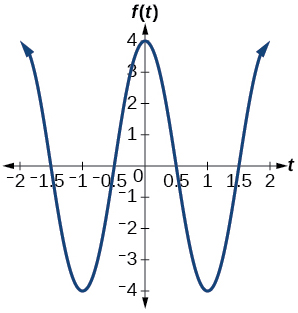amplitude: 4; period: 2; midline: $\text{\hspace{0.17em}}y=0;\text{\hspace{0.17em}}$ maximum: $\text{\hspace{0.17em}}y=4\text{\hspace{0.17em}}$ occurs at $\text{\hspace{0.17em}}x=0;\text{\hspace{0.17em}}$ minimum: $\text{\hspace{0.17em}}y=-4\text{\hspace{0.17em}}$ occurs at $\text{\hspace{0.17em}}x=1$

$f\left(x\right)=3\text{\hspace{0.17em}}\mathrm{cos}\left(\frac{6}{5}x\right)$

$y=3\text{\hspace{0.17em}}\mathrm{sin}\left(8\left(x+4\right)\right)+5$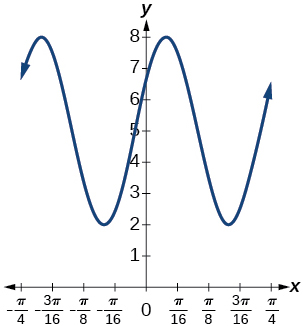amplitude: 3; period: $\text{\hspace{0.17em}}\frac{\pi }{4};\text{\hspace{0.17em}}$ midline: $\text{\hspace{0.17em}}y=5;\text{\hspace{0.17em}}$ maximum: $\text{\hspace{0.17em}}y=8\text{\hspace{0.17em}}$ occurs at $\text{\hspace{0.17em}}x=0.12;\text{\hspace{0.17em}}$ minimum: $\text{\hspace{0.17em}}y=2\text{\hspace{0.17em}}$ occurs at $\text{\hspace{0.17em}}x=0.516;\text{\hspace{0.17em}}$ horizontal shift: $\text{\hspace{0.17em}}-4;\text{\hspace{0.17em}}$ vertical translation 5; one period occurs from $\text{\hspace{0.17em}}x=0\text{\hspace{0.17em}}$ to $\text{\hspace{0.17em}}x=\frac{\pi }{4}$

$y=2\text{\hspace{0.17em}}\mathrm{sin}\left(3x-21\right)+4$

$y=5\text{\hspace{0.17em}}\mathrm{sin}\left(5x+20\right)-2$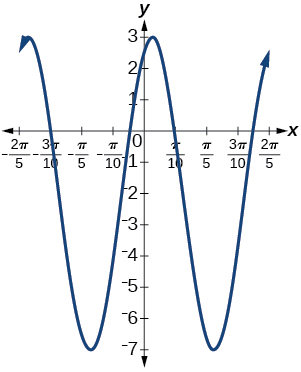amplitude: 5; period: $\text{\hspace{0.17em}}\frac{2\pi }{5};\text{\hspace{0.17em}}$ midline: $\text{\hspace{0.17em}}y=-2;\text{\hspace{0.17em}}$ maximum: $\text{\hspace{0.17em}}y=3\text{\hspace{0.17em}}$ occurs at $\text{\hspace{0.17em}}x=0.08;\text{\hspace{0.17em}}$ minimum: $\text{\hspace{0.17em}}y=-7\text{\hspace{0.17em}}$ occurs at $\text{\hspace{0.17em}}x=0.71;\text{\hspace{0.17em}}$ phase shift: $\text{\hspace{0.17em}}-4;\text{\hspace{0.17em}}$ vertical translation: $\text{\hspace{0.17em}}-2;\text{\hspace{0.17em}}$ one full period can be graphed on $\text{\hspace{0.17em}}x=0\text{\hspace{0.17em}}$ to $\text{\hspace{0.17em}}x=\frac{2\pi }{5}$

For the following exercises, graph one full period of each function, starting at $\text{\hspace{0.17em}}x=0.\text{\hspace{0.17em}}$ For each function, state the amplitude, period, and midline. State the maximum and minimum y -values and their corresponding x -values on one period for $\text{\hspace{0.17em}}x>0.\text{\hspace{0.17em}}$ State the phase shift and vertical translation, if applicable. Round answers to two decimal places if necessary.

$f\left(t\right)=2\mathrm{sin}\left(t-\frac{5\pi }{6}\right)$

$f\left(t\right)=-\mathrm{cos}\left(t+\frac{\pi }{3}\right)+1$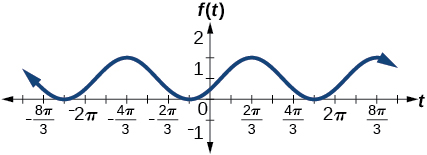amplitude: 1 ; period: $\text{\hspace{0.17em}}2\pi ;\text{\hspace{0.17em}}$ midline: $\text{\hspace{0.17em}}y=1;\text{\hspace{0.17em}}$ maximum: $\text{\hspace{0.17em}}y=2\text{\hspace{0.17em}}$ occurs at $\text{\hspace{0.17em}}x=2.09;\text{\hspace{0.17em}}$ maximum: $\text{\hspace{0.17em}}y=2\text{\hspace{0.17em}}$ occurs at $\text{\hspace{0.17em}}t=2.09;\text{\hspace{0.17em}}$ minimum: $\text{\hspace{0.17em}}y=0\text{\hspace{0.17em}}$ occurs at $\text{\hspace{0.17em}}t=5.24;\text{\hspace{0.17em}}$ phase shift: $\text{\hspace{0.17em}}-\frac{\pi }{3};\text{\hspace{0.17em}}$ vertical translation: 1; one full period is from $\text{\hspace{0.17em}}t=0\text{\hspace{0.17em}}$ to $\text{\hspace{0.17em}}t=2\pi$

$f\left(t\right)=4\mathrm{cos}\left(2\left(t+\frac{\pi }{4}\right)\right)-3$

$f\left(t\right)=-\mathrm{sin}\left(\frac{1}{2}t+\frac{5\pi }{3}\right)$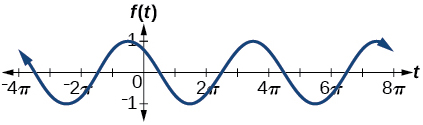amplitude: 1; period: $\text{\hspace{0.17em}}4\pi ;\text{\hspace{0.17em}}$ midline: $\text{\hspace{0.17em}}y=0;\text{\hspace{0.17em}}$ maximum: $\text{\hspace{0.17em}}y=1\text{\hspace{0.17em}}$ occurs at $\text{\hspace{0.17em}}t=11.52;\text{\hspace{0.17em}}$ minimum: $\text{\hspace{0.17em}}y=-1\text{\hspace{0.17em}}$ occurs at $\text{\hspace{0.17em}}t=5.24;\text{\hspace{0.17em}}$ phase shift: $\text{\hspace{0.17em}}-\frac{10\pi }{3};\text{\hspace{0.17em}}$ vertical shift: 0

$f\left(x\right)=4\mathrm{sin}\left(\frac{\pi }{2}\left(x-3\right)\right)+7$

Determine the amplitude, midline, period, and an equation involving the sine function for the graph shown in [link] .

amplitude: 2; midline: $\text{\hspace{0.17em}}y=-3;\text{\hspace{0.17em}}$ period: 4; equation: $\text{\hspace{0.17em}}f\left(x\right)=2\mathrm{sin}\left(\frac{\pi }{2}x\right)-3$

Determine the amplitude, period, midline, and an equation involving cosine for the graph shown in [link] .

Determine the amplitude, period, midline, and an equation involving cosine for the graph shown in [link] .

amplitude: 2; period: 5; midline: $\text{\hspace{0.17em}}y=3;\text{\hspace{0.17em}}$ equation: $\text{\hspace{0.17em}}f\left(x\right)=-2\mathrm{cos}\left(\frac{2\pi }{5}x\right)+3$

Determine the amplitude, period, midline, and an equation involving sine for the graph shown in [link] .

Determine the amplitude, period, midline, and an equation involving cosine for the graph shown in [link] .

amplitude: 4; period: 2; midline: $\text{\hspace{0.17em}}y=0;\text{\hspace{0.17em}}$ equation: $\text{\hspace{0.17em}}f\left(x\right)=-4\mathrm{cos}\left(\pi \left(x-\frac{\pi }{2}\right)\right)$

Determine the amplitude, period, midline, and an equation involving sine for the graph shown in [link] .

Determine the amplitude, period, midline, and an equation involving cosine for the graph shown in [link] .

amplitude: 2; period: 2; midline $\text{\hspace{0.17em}}y=1;\text{\hspace{0.17em}}$ equation: $\text{\hspace{0.17em}}f\left(x\right)=2\mathrm{cos}\left(\pi x\right)+1$

Determine the amplitude, period, midline, and an equation involving sine for the graph shown in [link] .

## Algebraic

For the following exercises, let $\text{\hspace{0.17em}}f\left(x\right)=\mathrm{sin}\text{\hspace{0.17em}}x.$

On $\text{\hspace{0.17em}}\left[0,2\pi \right),$ solve $\text{\hspace{0.17em}}f\left(x\right)=0.$

On $\text{\hspace{0.17em}}\left[0,2\pi \right),$ solve $\text{\hspace{0.17em}}f\left(x\right)=\frac{1}{2}.$

$\frac{\pi }{6},\frac{5\pi }{6}$

Evaluate $\text{\hspace{0.17em}}f\left(\frac{\pi }{2}\right).$

On $\text{\hspace{0.17em}}\left[0,2\pi \right),f\left(x\right)=\frac{\sqrt{2}}{2}.\text{\hspace{0.17em}}$ Find all values of $\text{\hspace{0.17em}}x.$

$\frac{\pi }{4},\frac{3\pi }{4}$

On $\text{\hspace{0.17em}}\left[0,2\pi \right),$ the maximum value(s) of the function occur(s) at what x -value(s)?

On $\text{\hspace{0.17em}}\left[0,2\pi \right),$ the minimum value(s) of the function occur(s) at what x -value(s)?

$\frac{3\pi }{2}$

Show that $\text{\hspace{0.17em}}f\left(-x\right)=-f\left(x\right).\text{\hspace{0.17em}}$ This means that $\text{\hspace{0.17em}}f\left(x\right)=\mathrm{sin}\text{\hspace{0.17em}}x\text{\hspace{0.17em}}$ is an odd function and possesses symmetry with respect to ________________.

For the following exercises, let $\text{\hspace{0.17em}}f\left(x\right)=\mathrm{cos}\text{\hspace{0.17em}}x.$

On $\text{\hspace{0.17em}}\left[0,2\pi \right),$ solve the equation $\text{\hspace{0.17em}}f\left(x\right)=\mathrm{cos}\text{\hspace{0.17em}}x=0.$

$\frac{\pi }{2},\frac{3\pi }{2}$

On $\text{\hspace{0.17em}}\left[0,2\pi \right),$ solve $\text{\hspace{0.17em}}f\left(x\right)=\frac{1}{2}.$

On $\text{\hspace{0.17em}}\left[0,2\pi \right),$ find the x -intercepts of $\text{\hspace{0.17em}}f\left(x\right)=\mathrm{cos}\text{\hspace{0.17em}}x.$

$\frac{\pi }{2},\frac{3\pi }{2}$

On $\text{\hspace{0.17em}}\left[0,2\pi \right),$ find the x -values at which the function has a maximum or minimum value.

On $\text{\hspace{0.17em}}\left[0,2\pi \right),$ solve the equation $\text{\hspace{0.17em}}f\left(x\right)=\frac{\sqrt{3}}{2}.$

$\frac{\pi }{6},\frac{11\pi }{6}$

## Technology

Graph $\text{\hspace{0.17em}}h\left(x\right)=x+\mathrm{sin}\text{\hspace{0.17em}}x\text{\hspace{0.17em}}$ on $\text{\hspace{0.17em}}\left[0,2\pi \right].\text{\hspace{0.17em}}$ Explain why the graph appears as it does.

Graph $\text{\hspace{0.17em}}h\left(x\right)=x+\mathrm{sin}\text{\hspace{0.17em}}x\text{\hspace{0.17em}}$ on $\text{\hspace{0.17em}}\left[-100,100\right].\text{\hspace{0.17em}}$ Did the graph appear as predicted in the previous exercise?

The graph appears linear. The linear functions dominate the shape of the graph for large values of $\text{\hspace{0.17em}}x.$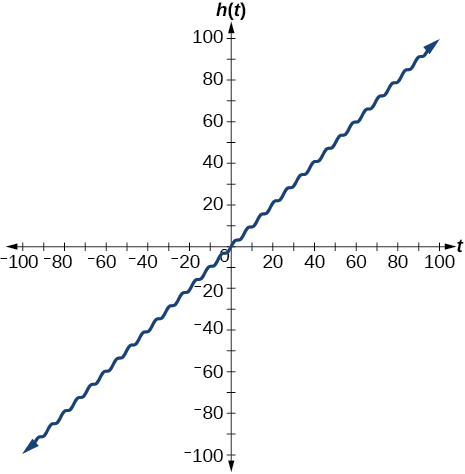Graph $\text{\hspace{0.17em}}f\left(x\right)=x\text{\hspace{0.17em}}\mathrm{sin}\text{\hspace{0.17em}}x\text{\hspace{0.17em}}$ on $\text{\hspace{0.17em}}\left[0,2\pi \right]\text{\hspace{0.17em}}$ and verbalize how the graph varies from the graph of $\text{\hspace{0.17em}}f\left(x\right)=\mathrm{sin}\text{\hspace{0.17em}}x.$

Graph $\text{\hspace{0.17em}}f\left(x\right)=x\text{\hspace{0.17em}}\mathrm{sin}\text{\hspace{0.17em}}x\text{\hspace{0.17em}}$ on the window $\text{\hspace{0.17em}}\left[-10,10\right]\text{\hspace{0.17em}}$ and explain what the graph shows.

The graph is symmetric with respect to the y -axis and there is no amplitude because the function is not periodic.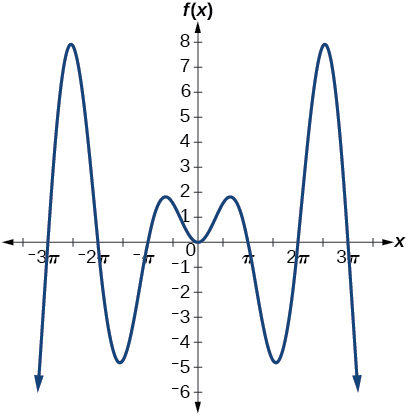Graph $\text{\hspace{0.17em}}f\left(x\right)=\frac{\mathrm{sin}\text{\hspace{0.17em}}x}{x}\text{\hspace{0.17em}}$ on the window $\text{\hspace{0.17em}}\left[-5\pi ,5\pi \right]\text{\hspace{0.17em}}$ and explain what the graph shows.

## Real-world applications

A Ferris wheel is 25 meters in diameter and boarded from a platform that is 1 meter above the ground. The six o’clock position on the Ferris wheel is level with the loading platform. The wheel completes 1 full revolution in 10 minutes. The function $\text{\hspace{0.17em}}h\left(t\right)\text{\hspace{0.17em}}$ gives a person’s height in meters above the ground t minutes after the wheel begins to turn.

1. Find the amplitude, midline, and period of $\text{\hspace{0.17em}}h\left(t\right).$
2. Find a formula for the height function $\text{\hspace{0.17em}}h\left(t\right).$
3. How high off the ground is a person after 5 minutes?
1. Amplitude: 12.5; period: 10; midline: $\text{\hspace{0.17em}}y=13.5;$
2. $h\left(t\right)=12.5\mathrm{sin}\left(\frac{\pi }{5}\left(t-2.5\right)\right)+13.5;$
3. 26 ft

#### Questions & Answers

A laser rangefinder is locked on a comet approaching Earth. The distance g(x), in kilometers, of the comet after x days, for x in the interval 0 to 30 days, is given by g(x)=250,000csc(π30x). Graph g(x) on the interval [0, 35]. Evaluate g(5)  and interpret the information. What is the minimum distance between the comet and Earth? When does this occur? To which constant in the equation does this correspond? Find and discuss the meaning of any vertical asymptotes.
Kaitlyn Reply
The sequence is {1,-1,1-1.....} has
amit Reply
circular region of radious
Kainat Reply
how can we solve this problem
Joel Reply
Sin(A+B) = sinBcosA+cosBsinA
Eseka Reply
Prove it
Eseka
Please prove it
Eseka
hi
Joel
June needs 45 gallons of punch. 2 different coolers. Bigger cooler is 5 times as large as smaller cooler. How many gallons in each cooler?
Arleathia Reply
7.5 and 37.5
Nando
find the sum of 28th term of the AP 3+10+17+---------
Prince Reply
I think you should say "28 terms" instead of "28th term"
Vedant
the 28th term is 175
Nando
192
Kenneth
if sequence sn is a such that sn>0 for all n and lim sn=0than prove that lim (s1 s2............ sn) ke hole power n =n
SANDESH Reply
write down the polynomial function with root 1/3,2,-3 with solution
Gift Reply
if A and B are subspaces of V prove that (A+B)/B=A/(A-B)
Pream Reply
write down the value of each of the following in surd form a)cos(-65°) b)sin(-180°)c)tan(225°)d)tan(135°)
Oroke Reply
Prove that (sinA/1-cosA - 1-cosA/sinA) (cosA/1-sinA - 1-sinA/cosA) = 4
kiruba Reply
what is the answer to dividing negative index
Morosi Reply
In a triangle ABC prove that. (b+c)cosA+(c+a)cosB+(a+b)cisC=a+b+c.
Shivam Reply
give me the waec 2019 questions
Aaron Reply

### Read also:

#### Get the best Algebra and trigonometry course in your pocket!

Source:  OpenStax, Algebra and trigonometry. OpenStax CNX. Nov 14, 2016 Download for free at https://legacy.cnx.org/content/col11758/1.6
Google Play and the Google Play logo are trademarks of Google Inc.

Notification Switch

Would you like to follow the 'Algebra and trigonometry' conversation and receive update notifications?ByByByByByBy